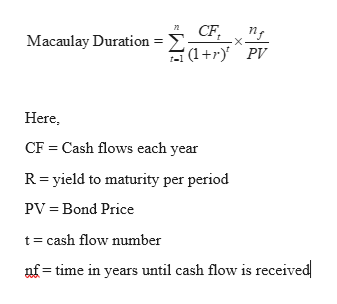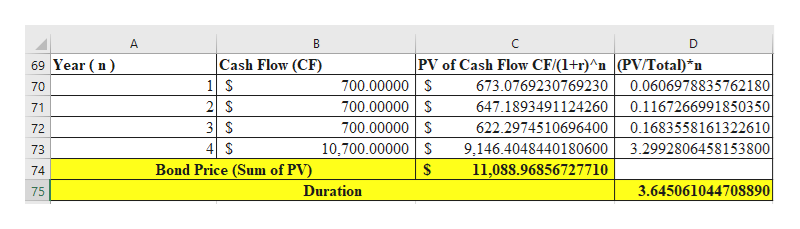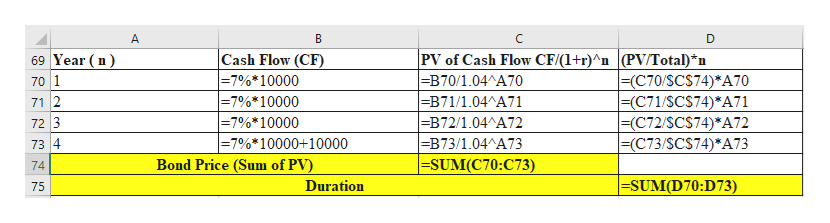# An organization purchased a four-year \$10,000 bond paying a 7% annual coupon and interest rates (for present value purposes are currently at 4%. This works out to a present value of the payment streams of \$673.08 + \$647.19 + \$622.30 + \$9,146.40 = \$11,088.97, which is the market price of the bond. What is the duration of this bond? 75 years65 years75 years0 years

Question
1 views
• An organization purchased a four-year \$10,000 bond paying a 7% annual coupon and interest rates (for present value purposes are currently at 4%. This works out to a present value of the payment streams of \$673.08 + \$647.19 + \$622.30 + \$9,146.40 = \$11,088.97, which is the market price of the bond. What is the duration of this bond?

1. 75 years
2. 65 years
3. 75 years
4. 0 years
check_circle

Step 1

Duration of a bond measures the interest rate sensitivity of the bond. However, Macaulay duration measures the number of years it takes to repay the investors the price of the bond. It takes the cash flows in the form of coupon and redemption value at the end of the maturity period.help_outlineImage Transcriptioncloseд CF, A(1 +r)^PV п, Macaulay Duration Here, CF = Cash flows each year R= yield to maturity per period PV = Bond Price t= cash flow number nf = time in years until cash flow is received fullscreen
Step 2

Duration of the bond is calculated as below:

Here, r = yield to maturity = 4%help_outlineImage TranscriptionclosePV of Cash Flow CF/(1+r)^n (PV/Total)*n 69 Year (n) Cash Flow (CF) 700.00000 S 700.00000 S 700.00000 S 10,700.00000 S 0.0606978835762180 0.1167266991850350 673.0769230769230 70 2 S 3 S 4 \$ Bond Price (Sum of PV) 647.1893491124260 71 622.2974510696400 0.1683558161322610 72 3.2992806458153800 9,146.4048440180600 73 11,088.96856727710 74 Duration 3.64506104470S890 75 fullscreen
Step 3

Excel wroki...help_outlineImage TranscriptionclosePV of Cash Flow CF/(1+r)^n (PV/Total)*n =B70/1.04^A7o =B71/1.04^A71 =B72/1.04^A72 =B73/1.04^A73 =SUM(C70:C73) 69 Year (n) Cash Flow (CF) =7%*10000 |=7%*10000 |=7%*10000 =7%*10000+10000 |=(C70/SC\$74)*A70 =(C71/SCS74)*A71 =(C72/SCS74)*A72 =(C73/SCS74)*A73 70 1 71 2 72 3 73 4 Bond Price (Sum of PV) 74 =SUM(D70:D73) Duration 75 fullscreen

### Want to see the full answer?

See Solution

#### Want to see this answer and more?

Solutions are written by subject experts who are available 24/7. Questions are typically answered within 1 hour.*

See Solution
*Response times may vary by subject and question.
Tagged in